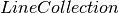Warning

This documents an unmaintained version of NetworkX. Please upgrade to a maintained version and see the current NetworkX documentation.

# draw_networkx_edges¶

draw_networkx_edges(G, pos, edgelist=None, width=1.0, edge_color='k', style='solid', alpha=1.0, edge_cmap=None, edge_vmin=None, edge_vmax=None, ax=None, arrows=True, label=None, **kwds)[source]

Draw the edges of the graph G.

This draws only the edges of the graph G.

Parameters: G (graph) – A networkx graph pos (dictionary) – A dictionary with nodes as keys and positions as values. Positions should be sequences of length 2. edgelist (collection of edge tuples) – Draw only specified edges(default=G.edges()) width (float, or array of floats) – Line width of edges (default=1.0) edge_color (color string, or array of floats) – Edge color. Can be a single color format string (default=’r’), or a sequence of colors with the same length as edgelist. If numeric values are specified they will be mapped to colors using the edge_cmap and edge_vmin,edge_vmax parameters. style (string) – Edge line style (default=’solid’) (solid|dashed|dotted,dashdot) alpha (float) – The edge transparency (default=1.0) cmap (edge) – Colormap for mapping intensities of edges (default=None) edge_vmin,edge_vmax (floats) – Minimum and maximum for edge colormap scaling (default=None) ax (Matplotlib Axes object, optional) – Draw the graph in the specified Matplotlib axes. arrows (bool, optional (default=True)) – For directed graphs, if True draw arrowheads. label ([None| string]) – Label for legendof the edges matplotlib.collection.LineCollection

Notes

For directed graphs, “arrows” (actually just thicker stubs) are drawn at the head end. Arrows can be turned off with keyword arrows=False. Yes, it is ugly but drawing proper arrows with Matplotlib this way is tricky.

Examples

>>> G=nx.dodecahedral_graph()
>>> edges=nx.draw_networkx_edges(G,pos=nx.spring_layout(G))


Also see the NetworkX drawing examples at https://networkx.org/documentation/latest/gallery.html